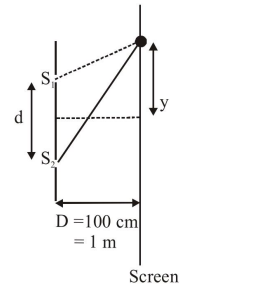# Interference fringes are observed on a screen by illuminating two thin slits

Question:

Interference fringes are observed on a screen by illuminating two thin slits $1 \mathrm{~mm}$ apart with a light source $(\lambda=632.8 \mathrm{~nm})$. The distance between the screen and the slits is $100 \mathrm{~cm}$. If a bright fringe is observed on a screen at a distance of $1.27 \mathrm{~mm}$ from the central bright fringe, then the path difference between the waves, which are reaching this point from the slits is close to :

1. $1.27 \mu \mathrm{m}$

2. $2 \mathrm{~nm}$

3. $2.87 \mathrm{~nm}$

4. $2.05 \mu \mathrm{m}$

Correct Option: 1

Solution:$\mathrm{y}=\frac{\mathrm{n} \mathrm{D} \lambda}{\mathrm{d}}$

$\mathrm{n}=\frac{\mathrm{yd}}{\mathrm{D} \lambda}=\frac{1.27 \times 10^{-3} \times 10^{-3}}{1 \times 632.8 \times 10^{-9}}=2$

Path difference $\Delta x=n \lambda$

$=2 \times 632.8 \mathrm{~nm}$

$=1265.6 \mathrm{~nm}$

$=1.27 \mu \mathrm{m}$